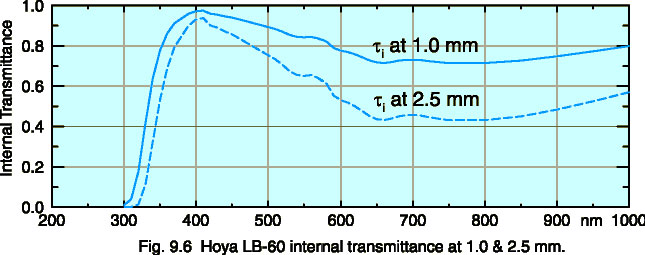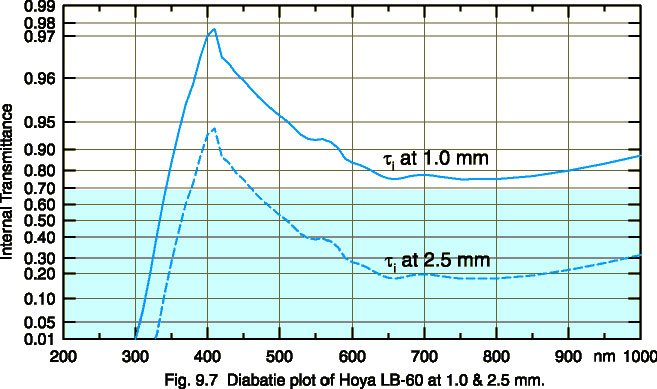### GRAPHING DATA

Line Sources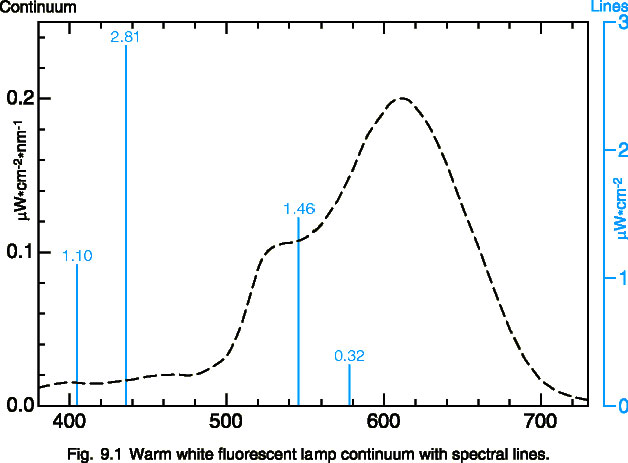Discharge sources emit large amounts of irradiance at particular atomic spectral lines, in addition to a constant, thermal based continuum. The most accurate way to portray both of these aspects on the same graph is with a dual axis plot, shown in figure 9.1. The spectral lines are graphed on an irradiance axis (W/cm2) and the continuum is graphed on a band irradiance (W/cm2/nm) axis. The spectral lines ride on top of the continuum.

Another useful way to graph mixed sources is to plot spectral lines as a rectangle the width of the monochromator bandwidth. (see fig. 5.6) This provides a good visual indication of the relative amount of power contributed by the spectral lines in relation to the continuum, with the power being bandwidth times magnitude.

Polar Spatial Plots

The best way to represent the responsivity of a detector with respect to incident angle of light is by graphing it in Polar Coordinates. The polar plot in figure 9.2 shows three curves: A power response (such as a laser beam under filling a detector), a cosine response (irradiance overfilling a detector), and a high gain response (the effect of using a telescopic lens). This method of graphing is desirable, because it is easy to understand visually. Angles are portrayed as angles, and responsivity is portrayed radially in linear graduations.

The power response curve clearly shows that the response between -60 and +60 degrees is uniform at 100 percent. This would be desirable if you were measuring a laser or focused beam of light, and underfilling a detector. The uniform response means that the detector will ignore angular misalignment

The cosine response is shown as a circle on the graph. An irradiance detector with a cosine spatial response will read 100 percent at 0 degrees (straight on), 70.7 percent at 45 degrees, and 50 percent at 60 degrees incident angle. (Note that the cosines of 0°, 45° and 60°, are 1.0, 0.707, and 0.5, respectively).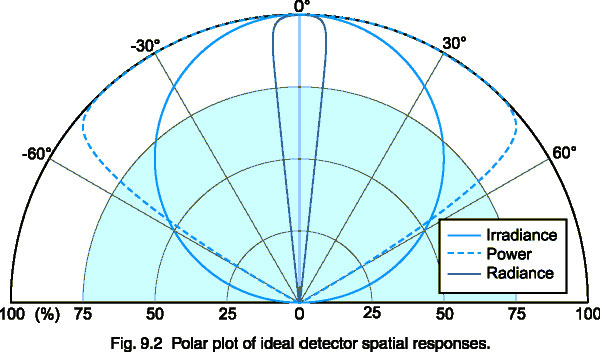The radiance response curve has a restricted field of view of ± 5°. Many radiance barrels restrict the field of view even further (± 1-2° is common). High gain lenses restrict the field of view in a similar fashion, providing additional gain at the expense of lost off angle measurement capability.

Cartesian Spatial Plots

The cartesian graph in figure 9.3 contains the same data as the polar plot in figure 9.2 on the previous. The power and high gain curves are fairly easy to interpret, but the cosine curve is more difficult to visually recognize. Many companies give their detector spatial responses in this format, because it masks errors in the cosine correction of the diffuser optics. In a polar plot the error is easier to recognize, since the ideal cosine response is a perfect circle.

In full immersion applications such as phototherapy, where light is coming from all directions, a cosine spatial response is very important. The skin (as well as most diffuse, planar surfaces) has a cosine response. If a cosine response is important to your application, request spatial response data in polar format.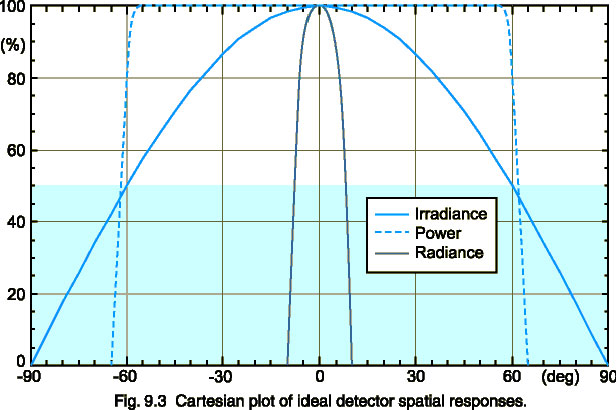At the very least, the true cosine response should be superimposed over the Cartesian plot of spatial response to provide some measure of comparison.

Note: Most graphing software packages do not provide for the creation of polar axes. Microsoft Excel, for example, does have "radar" category charts, but does not support polar scatter plots yet. SigmaPlot, an excellent scientific graphing package, supports polar plots, as well as custom axes such as log-log etc. (Ref: http://www.spss.com/software/science/sigmaplot)

Logarithmically Scaled Plots

A log plot portrays each 10 to 1 change as a fixed linear displacement. Logarithmically scaled plots are extremely useful at showing two important aspects of a data set. First, the log plot expands the resolution of the data at the lower end of the scale to portray data that would be difficult to see on a linear plot. The log scale never reaches zero, so data points that are 1 millionth of the peak still receive equal treatment. On a linear plot, points near zero simply disappear.

The second advantage of the log plot is that percentage difference is represented by the same linear displacement everywhere on the graph. On a linear plot, 0.09 is much closer to 0.10 than 9 is to 10, although both sets of numbers differ by exactly 10 percent. On a log plot, 0.09 and 0.10 are the same distance apart as 9 and 10, 900 and 1000, and even 90 billion and 100 billion. This makes it much easier to determine a spectral match on a log plot than a linear plot.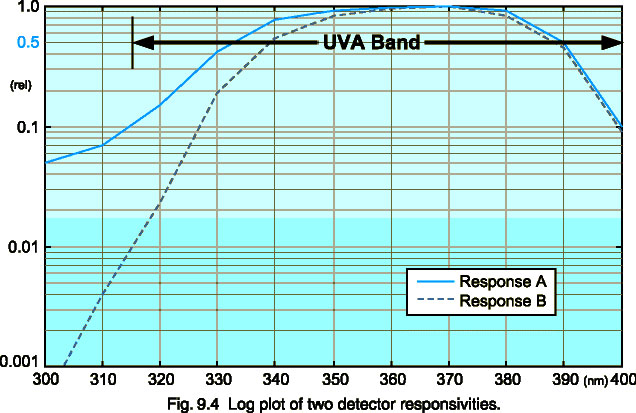As you can clearly see in figure 9.4, response B is within 10 percent of response A between 350 and 400 nm. Below 350 nm, however, they clearly mismatch. In fact, at 315 nm, response B is 10 times higher than response A. This mismatch is not evident in the linear plot, figure 9.5, which is plotted with the same data.

One drawback of the log plot is that it compresses the data at the top end, giving the appearance that the bandwidth is wider than it actually is. Note that Figure 9.4 appears to approximate the UVA band.

Linearly Scaled Plots

Most people are familiar with graphs that utilize linearly scaled axes. This type of graph is excellent at showing bandwidth, which is usually judged at the 50 percent power points. In figure 9.5, it is easy to see that response A has a bandwidth of about 58 nm (332 to 390 nm). It is readily apparent from this graph that neither response A nor response B would adequately cover the entire UVA band (315 to 400 nm), based on the location of the 50 percent power points. In the log plot of the same data (fig. 9.4), both curves appear to fit nicely within the UVA band.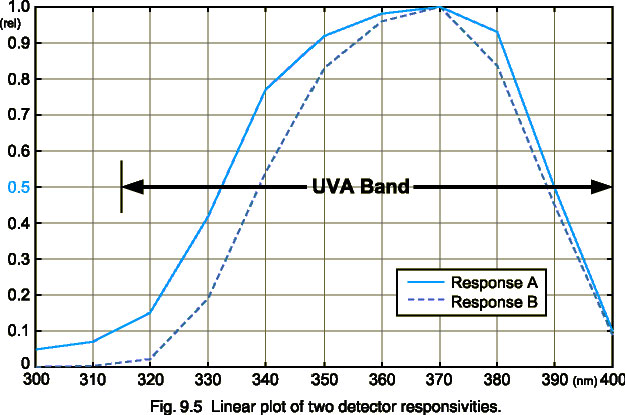This type of graph is poor at showing the effectiveness of a spectral match across an entire function. The two responses in the linear plot appear to match fairly well. Many companies, in an attempt to portray their products favorably, graph detector responses on a linear plot in order to make it seem as if their detector matches a particular photo-biological action spectrum, such as the Erythemal or Actinic functions. As you can clearly see in the logarithmic curve (fig. 9.4), response A matches response B fairly well above 350 nm, but is a gross mismatch below that. Both graphs were created from the same set of data, but convey a much different impression.

As a rule of thumb - half power bandwidth comparisons and peak spectral response should be presented on a linear plot. Spectral matching should be evaluated on a log plot.

Linear vs. Diabatie Spectral Transmission Curves

The Diabatie scale (see fig. 9.7) is a log-log scale used by filter glass manufacturers to show internal transmission for any thickness. The Diabatie value, q(l), is defined as follows according to DIN 1349:

q(l) = 1 - log(log(1/t))

Linear transmission curves are only useful for a single thickness (fig. 9.6). Diabatie curves retain the same shape for every filter glass thickness, permitting the use of a transparent sliding scale axis overlay, usually provided by the glass manufacturer. You merely line up the key on the desired thickness and the transmission curve is valid.